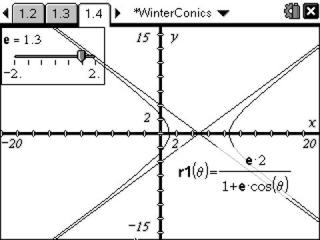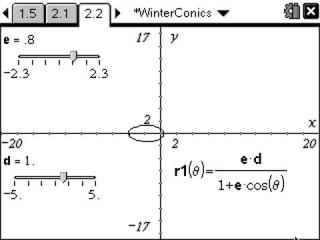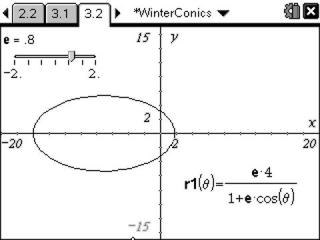# Activities

•##### Download

•• ##### Subject Area

• Math: Precalculus: Analytic Geometry (Conics)
• Math: Precalculus: Polar Equations

• ##### Author9-12

60 Minutes

• ##### Device
• TI-Nspire™ Navigator™
• TI-Nspire™
• TI-Nspire™ CAS
• ##### Software

TI-Nspire™
TI-Nspire™ CAS

2.0

• ##### Report an Issue

Conics In Winter#### Activity Overview

Students explore conic graphing using a polar notation equation and determine the effects the various variables on the graph.

#### Key Steps

•Students explore how the eccentricity affects the graph of a conic section in polar coordinates. They drag the pointer on a slider to change the value of the eccentricity and observe what happens to the graph.

They will see that for a parabola the eccentricity is 1, for a hyperbola the absolute value of the eccentricity is greater than 1, and the absolute value of the eccentricity of an ellipse is less than 1.

•Students go further by exploring the variable d. d represents the distance from the conic section to the directrix. They see how d affects different conic sections. They should also change the value of e and then change the value of d again.

•Students observe how other changes with the equation will affect the graph. They edit the equation and observe what happens when the plus sign becomes a minus sign and what happens when cosine becomes sine.

They see that the plus/minus sign reflects the graph about the y-axis. Using cosine results in the graph having a horizontal orientation, and sine results in a vertical orientation.